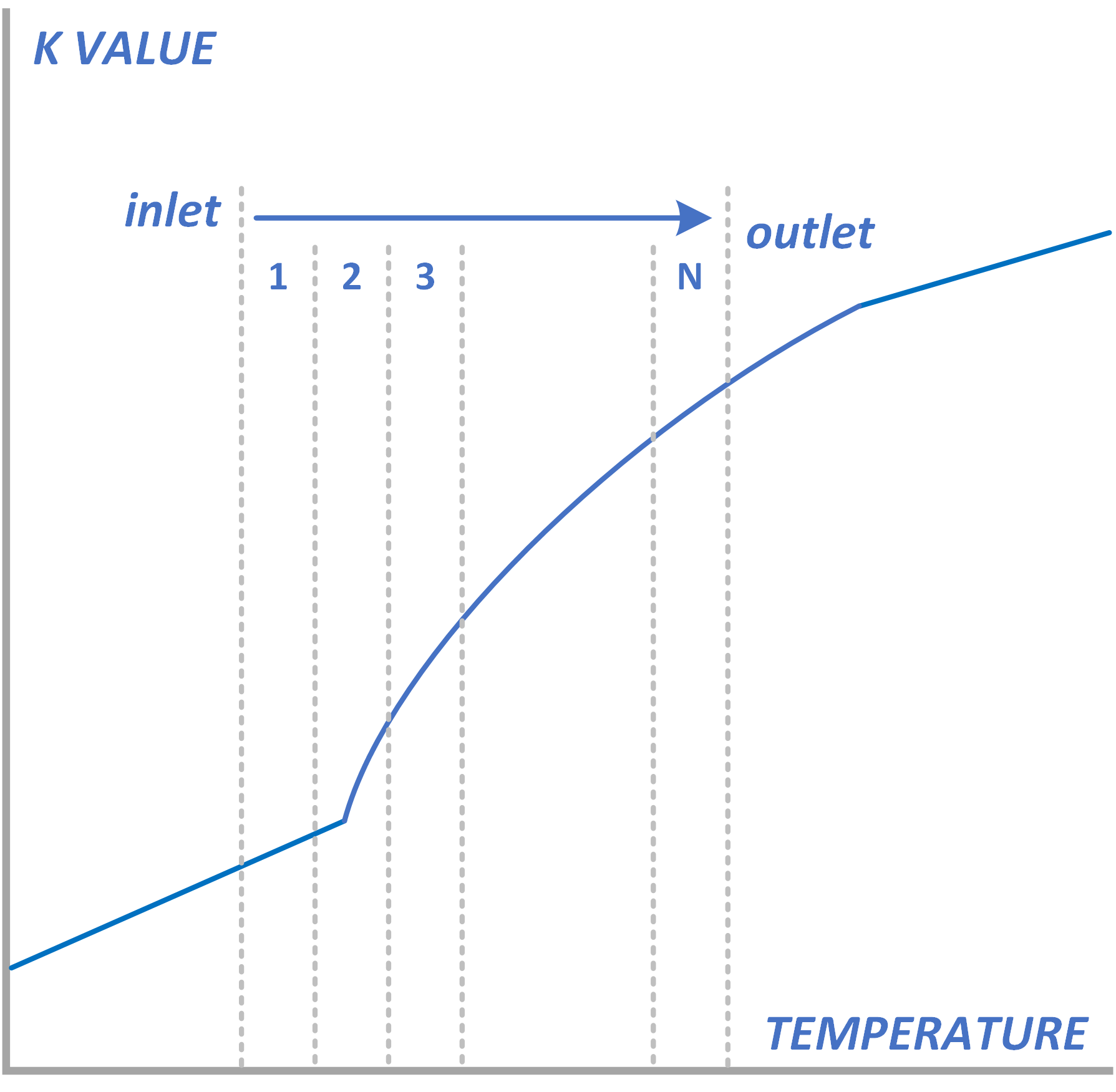AHED has been equipped with a procedure that reduces the error in the calculation for fluid flow that passes through the transition flow regime. Any switch from laminar to transition flow or from transition flow to turbulent flow usually sees some large changes in the rate of heat transfer. Traditional calculation methods don't take this phenomena too seriously and this can lead to errors in the final result.  The AHED-OPT calculation routine is included in the program to minimise this error as much as possible.

• The physical properties for shell and tube side fluids are calculated with the midpoint method. They are taken as an average of the properties at the inlet and outlet conditions. The heat transfer coefficient for shell and tube side and overall heat transfer coefficient are calculated based on the midpoint information only.
• The heat transfer coefficients are calculated at the inlet and outlet conditions and an average overall heat transfer coefficient is calculated based on these inlet and outlet values.

It will take much more of the installed heat transfer area to deal with the part that flows in laminar/transition flow than what is needed for the turbulent flow part. To correctly calculate this phenomena, the heat exchanger calculation should be divided up in many little parts and an individual heat transfer and area calculation should be done for each part. At the end, the sum of all parts will define the total heat transfer area that is needed. This is exactly what AHED does in a very clever way:

1. The first step is to calculate the heat transfer coefficient at the inlet and outlet. These values are averaged and the area that is needed is calculated based on this value.
2. Next, the heat exchanger temperature trajectory is divided in two halves. Like in step 1, the heat transfer coefficients are calculated for inlet and outlet condition of each part. T-start to T-50% and T-50% to T-end. For each half, the area is calculated based on the average of the two heat transfer coefficients at inlet and outlet of each half. The total area is the sum of the half-areas.
3. If the difference between the two areas calculated in step 1 and 2 is not within a predefined error limit, the heat exchanger is divided in four sections: T-start to T-25%, T-25% to T-50%, T-50% to T-75% and T-75% to T-end. Now we calculate the heat transfer and area for each of the four section and we sum to obtain the total area. We compare the total area with the value obtained in step 2 to check if convergence is obtained.
4. This process is repeated until the area in step N minus the area in step N-1 is below the error limit set.

Under normal conditions, after a few iterations, the calculation converges. For every heat transfer calculation AHED-OPT is run to make sure that the most optimal result is obtained always.

Released:
Created: